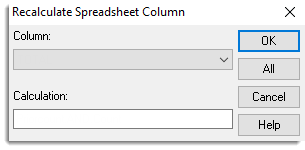1. Home
2. Recalculate Column

# Recalculate Column

This dialog lists the columns within a spreadsheet that have been created using a calculation. Columns can be calculated using a calculation menu. This dialog allows the calculation to be changed, and then rerun to recreate the column values. For example, if column C was generated as the sum of columns A and B, and if some values in either column A or B were changed, the new sum could be calculated and put into column C. Alternatively, if you wanted to add in another column (D) into the sum the calculation could be changed to A+B+D.

A column that has been calculated can also be displayed using the Column Attributes/Format dialog.## Column

The column to recalculate. The dropdown list contains columns which have been calculated using one of calculate menus, for example Calculate, Form Groups or Generate Random Sample.

## Calculation

The calculation that was used to create the column. You can change the calculation before recalculating the column.

## OK

Recreate the column via the calculation displayed.

## All

Recalculate all calculated columns in the spreadsheet.

## Cancel

Close the dialog and do not make any changes.